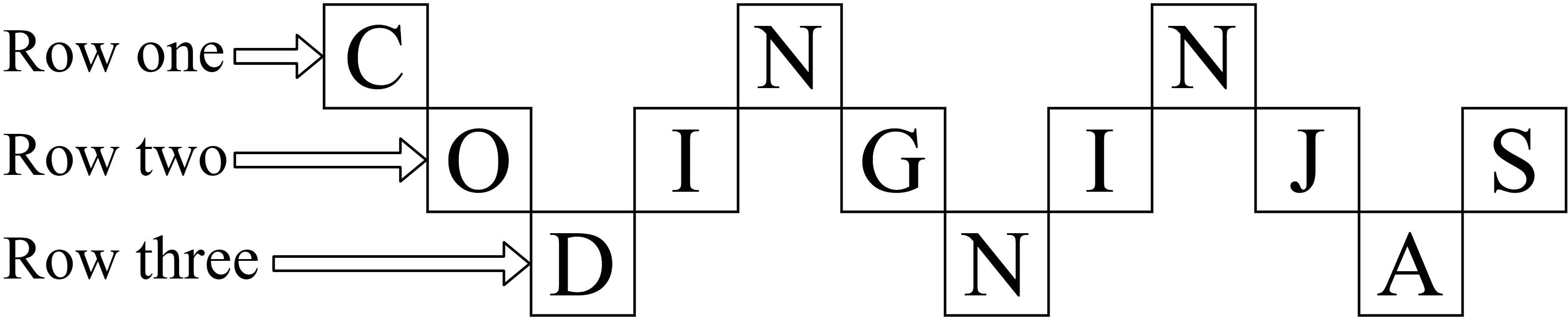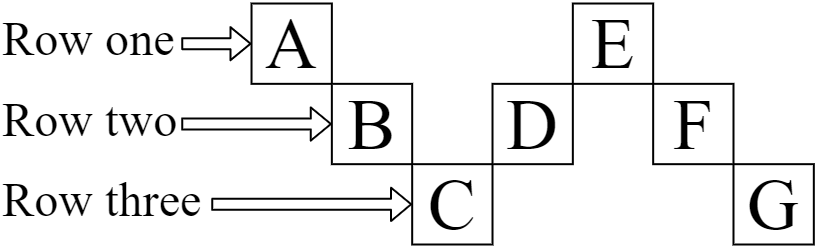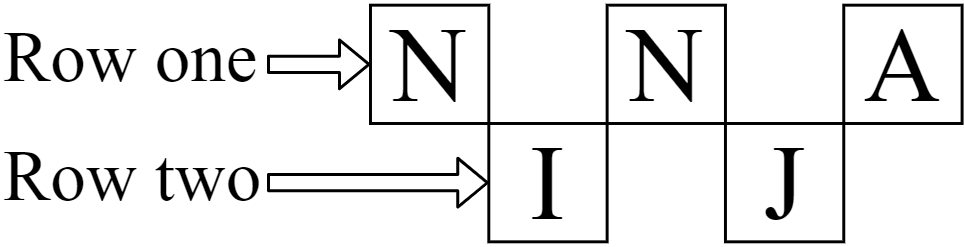1

# Zig-Zag String

Difficulty: EASY
Avg. time to solve
15 min
Success Rate
85%

Problem Statement
Suggest Edit

#### You are given a string ‘STR’ of size ‘N’ and an integer ‘M’ (the number of rows in the zig-zag pattern of ‘STR’). Your task is to return the string formed by concatenating all ‘M’ rows when string ‘STR’ is written in a row-wise zig-zag pattern.

##### Example:
``````N = 12, M = 3 and STR = ‘CODINGNINJAS’
````````````There are three rows (‘M = 3’) in the zig-zag pattern. Row one contains ‘CNN’, row two contains ‘OIGIJS’, and row three contains ‘DNA’. After concatenating the three rows, we get the string ‘CNNOIGIJSDNA’. So, the answer is ‘CNNOIGIJSDNA’.
``````
##### Note:
``````1. The string ‘STR’ consists of capital letters only (i.e., characters from ‘A-Z’).
``````
##### Input format:
``````The first line of input contains an integer ‘T’ denoting the number of test cases.

The first line of each test case contains two space-separated integers, ‘N’ and ‘M’, denoting the size of string ‘STR’ and the number of rows in the zig-zag pattern, respectively.

The second line of each test case contains a string ‘STR’.
``````
##### Output format:
``````For each test case, return the string formed by concatenating all ‘M’ rows when string ‘STR’ is written in a zig-zag pattern.
``````
##### Note:
``````You do not need to print anything; it has already been taken care of. Just implement the function
``````
##### Constraints:
``````1 <= T <= 10^2
1 <= N <= 10^3
1 <= M <= N
‘STR’ contains only ‘A-Z’ characters.

Time Limit: 1 second
``````

#### Sample Input 1:

``````2
7 3
ABCDEFG
5 2
NINJA
``````

#### Sample Output 1:

``````AEBDFCG
NNAIJ
``````

#### Explanation For Sample Input 1:

``````Test Case 1:
````````````There are three rows (‘m = 3’) in the zig-zag pattern. Row one contains ‘AE’, row two contains ‘BDF’, and row three contains ‘CG’. After concatenating the three rows, we get the string ‘AEBDFCG’. So, the answer is ‘AEBDFCG’.

Test Case 2:
````````````There are two rows (‘m = 2’) in the zig-zag pattern. Row one contains ‘NNA’, and row two contains ‘IJ’. After concatenating the two rows, we get the string ‘NNAIJ’. So, the answer is ‘NNAIJ’.
``````

#### Sample Input 2:

``````2
4 2
PQRS
6 6
ZIGZAG
``````

#### Sample Output 2:

``````PRQS
ZIGZAG
``````Console